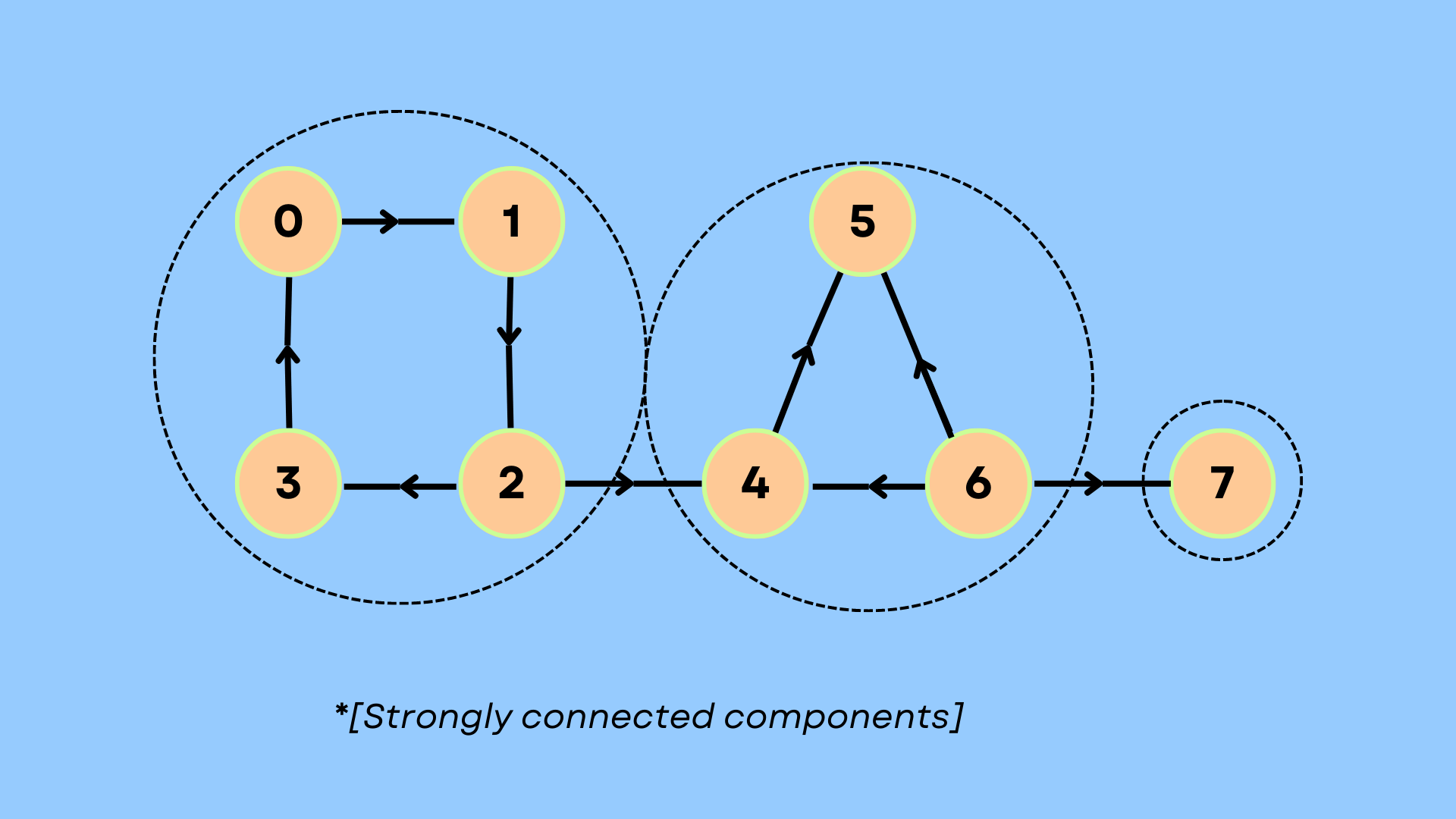# Biconnected Graph Algorithm with C++## Overview

In this article, we shall learn about biconnected graphs. They’re a kind of graph that is connected and have zero articulation points.

## Scope

• What is a biconnected graph?
• An algorithm to find if a graph is biconnected.
• Code implementation and examples.

## What is a biconnected graph?

If a graph has a path between any 2 different nodes, the graph is said to be connected. The following graph is connected since one vertex can be visited from any other vertex and at least one path exists between every pair of vertices. A node in the graph is said to be an articulation point if removing it as well as its edges becomes disconnected. Any graph that is connected and has no articulation points is said to be biconnected. The graph as shown in the diagram ahead is connected:

Following is an example of a graph that is not biconnected:

## Algorithm

• Given a connected undirected graph G. We try to find all the biconnected components of G by using depth-first search to find the depth-first spanning trees.
• We use a vector named dfn to preserve the order of the nodes in which they were explored.
• In a depth-first spanning tree dfn(v) > dfn(u), for each pair of nodes (u, v) such that u is an ancestor to v.
• We call an edge {x, y} a back-edge iff either exactly one of u and v is an ancestor to the other. Every non-tree edge is a back-edge.
• To determine if a node ‘v’ is an articulation point:
• The node is root: v will be an articulation point if it has a minimum of 2 children.
• The node is not a root: v will be an articulation point if it has a child ‘u’ such that in the tree rooted at u there does not exist any back-edge to any ancestor of v.
• We use a vector named low to keep track of the information related to the node that has the smallest discovery time and is accessible from a given vertex, utilizing up to a single back-edge.
• For each edge, we find the lowest value from “low” corresponding to the node.
 low[node] = min(low[child], low[node])
• If this edge is a back-edge, then:
 low[node] = min(discoveryTime[child], low[node])
• For any given node ‘u’ we must compare its depth-first number with the low value of a child ‘v’. If the low value is found to be higher than or the same as the depth-first number or the discovery time of u, then it is an articulation point.
 dfn[v] < low[u] || dfn[node] < low[child]
• We should now determine whether or not all the nodes that are reachable from a given node in the graph, are also accessible from every other node from the graph as well, on the removal of the given node.

## Implementation

 #include "iostream"#include "vector"#include "limits.h"#include "math.h"#include "algorithm"#include "functional"using namespace std;vector>g;void dfsMod(int u, vector& dfn, vector& low, vector& par, vector& cutVertices, vector& vis, int& time){    int childrenU = 0;    vis[u] = 1;    low[u] = dfn[u] = time++;        for(int child : g[u]){        if(dfn[child] == -1){            par[child] = u;            childrenU++;            dfsMod(child, dfn, low, par, cutVertices, vis, time);            low[u] = min(low[u], low[child]);                        if (par[u] == -1 && childrenU > 1){ cutVertices[u] = 1; } else if (par[u] != -1 && low[child] >= dfn[u]){ cutVertices[u] = 1; }        }        else if(par[u] != child){            low[u] = min(low[u], dfn[child]);        }    }}void cutVertices(int n, int e){    vectorpar(n+1, -1), dfn(n+1, -1), low(n+1, -1), cutVertices(n + 1), vis(n+1); int con = 1, ap = 0, time = 1; for (int i = 0; i < n; ++i){ if (dfn[i] == -1){ dfsMod(i, dfn, low, par, cutVertices, vis, time); time = 1; } } for(int i = 0;i < n;i++){        if(!vis[i]){            cout << "The graph is disconnected!";            con = 0;        }        ap+=(cutVertices[i]==1);    }    if(ap){        cout<<"The graph has articulation points:"<>n>>e;    g.resize(n);    for(int i=0;i>u>>v;        g[u].push_back(v), g[v].push_back(u);    }    cutVertices(n, e);}

Input

 3 20 11 2

Output

 The graph has articulation points:1
write your code here: Coding Playground